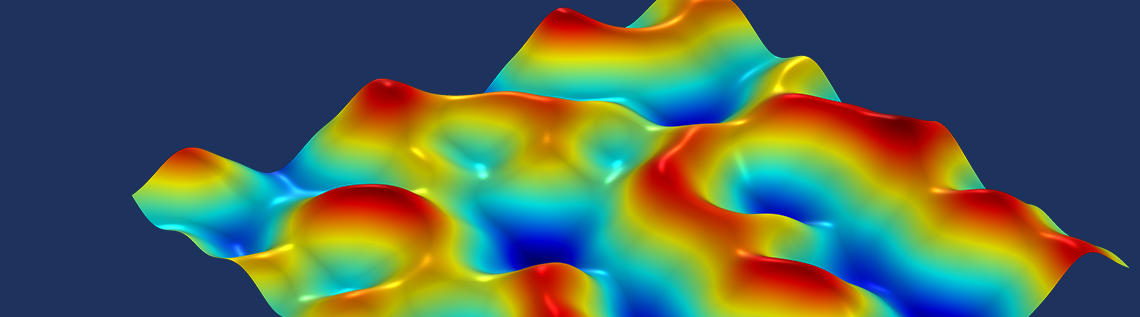# 分形、噪声和状态变量

2021年 1月 5日

### 什么是分形？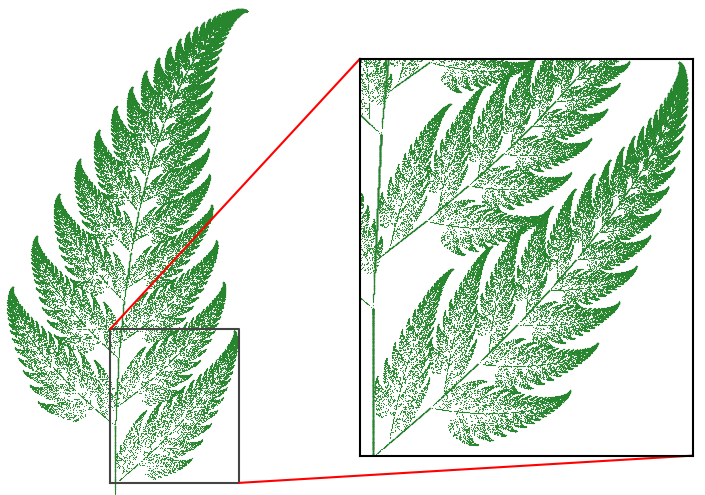### 芒德布罗集合

z_\textrm{n+1} = z_\textrm{n}^2 + c \qquad \textrm{其中} \qquad z_0 = 0

### 在 COMSOL Multiphysics® 中计算芒德布罗集合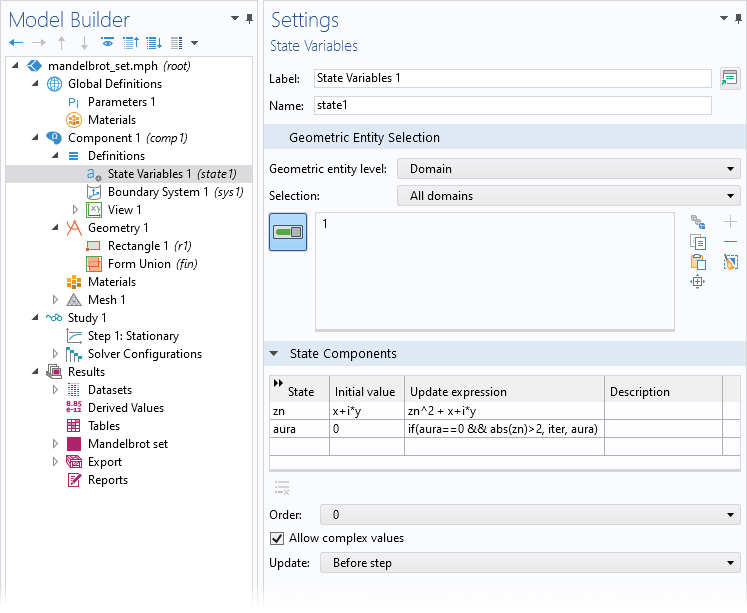### 分形噪声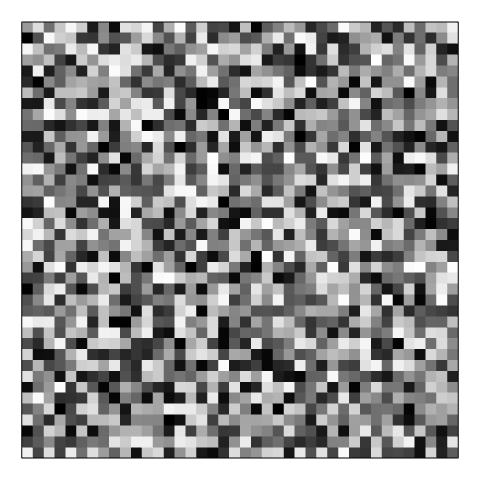#### 佩林噪声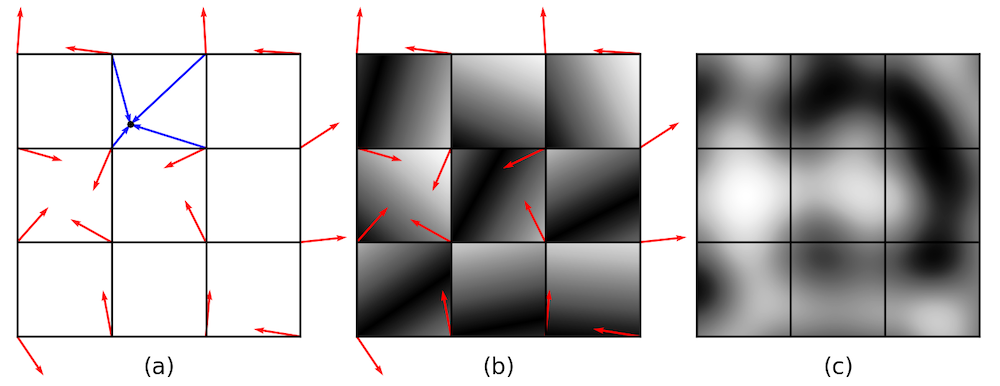d_\textrm{i} = \mathbf g_\textrm{i} \cdot (\mathbf x – \mathbf x_\textrm{gi}) \qquad \textrm{其中} \qquad i = 1,2,3,4

#### 分形噪声

h(x, y) = \sum_{j=0}^N \frac{\texttt{noise}(2^j x, \ 2^j y)}{\texttt{scale}(j)}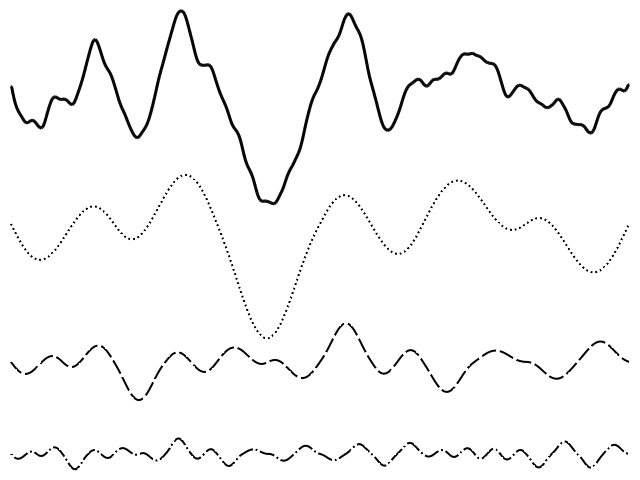### 自己尝试

1. K. Perlin, “Improving Noise”, New York University, 2002. https://mrl.cs.nyu.edu/~perlin/paper445.pdf.